# diff.c File Reference

The diff command source file. More...

`#include <stdlib.h>`
`#include <unistd.h>`
`#include <poll.h>`
`#include <alloca.h>`
`#include <time.h>`
`#include <fcntl.h>`
`#include "global.h"`
`#include "revert.h"`
`#include "helper.h"`
`#include "interface.h"`
`#include "url.h"`
`#include "status.h"`
`#include "options.h"`
`#include "est_ops.h"`
`#include "ignore.h"`
`#include "waa.h"`
`#include "racallback.h"`
`#include "cp_mv.h"`
`#include "warnings.h"`
`#include "diff.h"`
Include dependency graph for diff.c: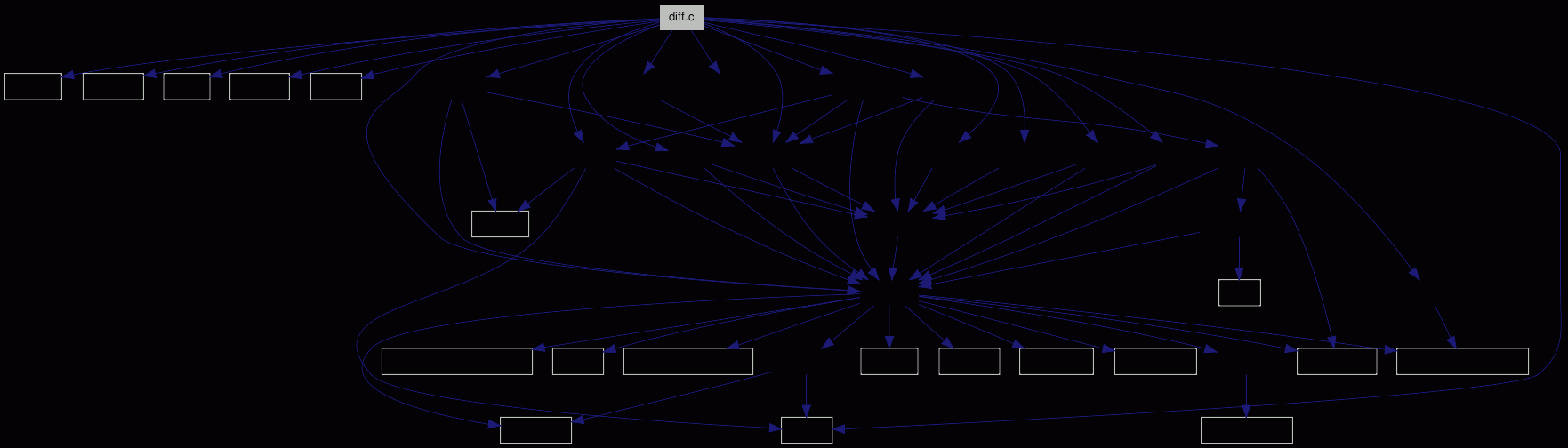Go to the source code of this file.

## Defines

#define META_DIFF_DELIMITER   (0xf44fee31)
A number that cannot be a valid pointer.
#define META_DIFF_MAXLEN   (256)
How long may a meta-data diff string be?

## Functions

int df___print_meta (char *format,...)
Diff the given meta-data.
int df__do_diff (struct estat *sts, svn_revnum_t rev1, svn_revnum_t rev2, char *rev2_file)
Get a file from the repository, and initiate a diff.
int df___cleanup (void)
Cleanup rests.
static void df___signal (int sig)
FSVS GCOV MARK: df___signal should not be executed.
int df___type_def_diff (struct estat *sts, svn_revnum_t rev, apr_pool_t *pool)
Does a diff of the local non-directory against the given revision.
int df___direct_diff (struct estat *sts)
-.
int df___cheap_colordiff (void)
A cheap replacement for colordiff.
int df___colordiff (int *handle, pid_t *cd_pid)
Tries to start colordiff.
int df___diff_wc_remote (struct estat *entry, apr_pool_t *pool)
Prints diffs for all entries with estat::entry_status or estat::remote_status set.
int df___reset_remote_st (struct estat *sts)
Set the entry as BASE (has no changes).
int df___repos_repos (struct estat *sts)
Does a repos/repos diff.
int df__work (struct estat *root, int argc, char *argv[])
-.

## Variables

int cdiff_pipe = STDOUT_FILENO
pid_t cdiff_pid = 0

## Detailed Description

The diff command source file.

Currently only diffing single files is possible; recursive diffing of trees has to be done.

For trees it might be better to fetch all files in a kind of update-scenario; then we'd avoid the many round-trips we'd have with single-file-fetching. Although an optimized file-fetching (rsync-like block transfers) would probably save a lot of bandwidth.

Definition in file diff.c.

## Define Documentation

 #define META_DIFF_DELIMITER   (0xf44fee31)

A number that cannot be a valid pointer.

Definition at line 92 of file diff.c.

Referenced by df___print_meta(), and df__do_diff().

 #define META_DIFF_MAXLEN   (256)

How long may a meta-data diff string be?

Definition at line 94 of file diff.c.

Referenced by df___print_meta().

## Function Documentation

 int df___cheap_colordiff ( void )

A cheap replacement for colordiff.

Nothing more than a `cat`.

Definition at line 582 of file diff.c.

References STOPIF_CODE_ERR.

Referenced by df___colordiff().

Here is the caller graph for this function: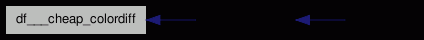int df___cleanup ( void )

Cleanup rests.

Definition at line 411 of file diff.c.

References cdiff_pid, cdiff_pipe, DEBUGP, df__do_diff(), STOPIF, and STOPIF_CODE_ERR.

Referenced by df___signal(), and df__work().

Here is the call graph for this function: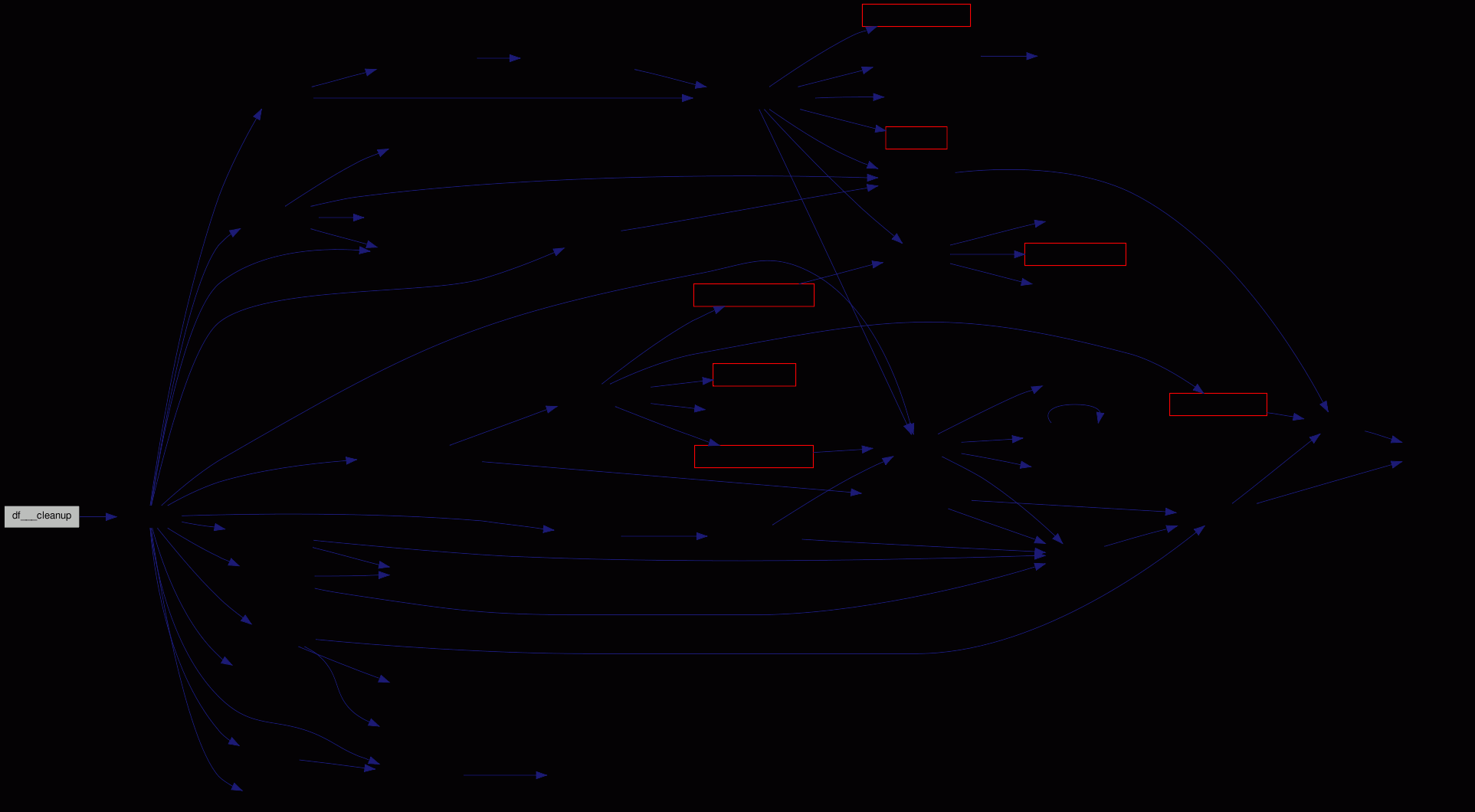Here is the caller graph for this function:int df___colordiff ( int * handle, pid_t * cd_pid )

Tries to start colordiff.

If colordiff can not be started, but the option says `auto`, we just forward the data. Sadly neither `splice` nor `sendfile` are available everywhere.

Definition at line 611 of file diff.c.

Referenced by df__work().

Here is the call graph for this function: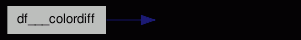Here is the caller graph for this function: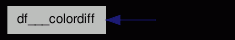int df___diff_wc_remote ( struct estat * entry, apr_pool_t * pool )

Prints diffs for all entries with estat::entry_status or estat::remote_status set.

Definition at line 719 of file diff.c.

Referenced by df__work().

Here is the call graph for this function: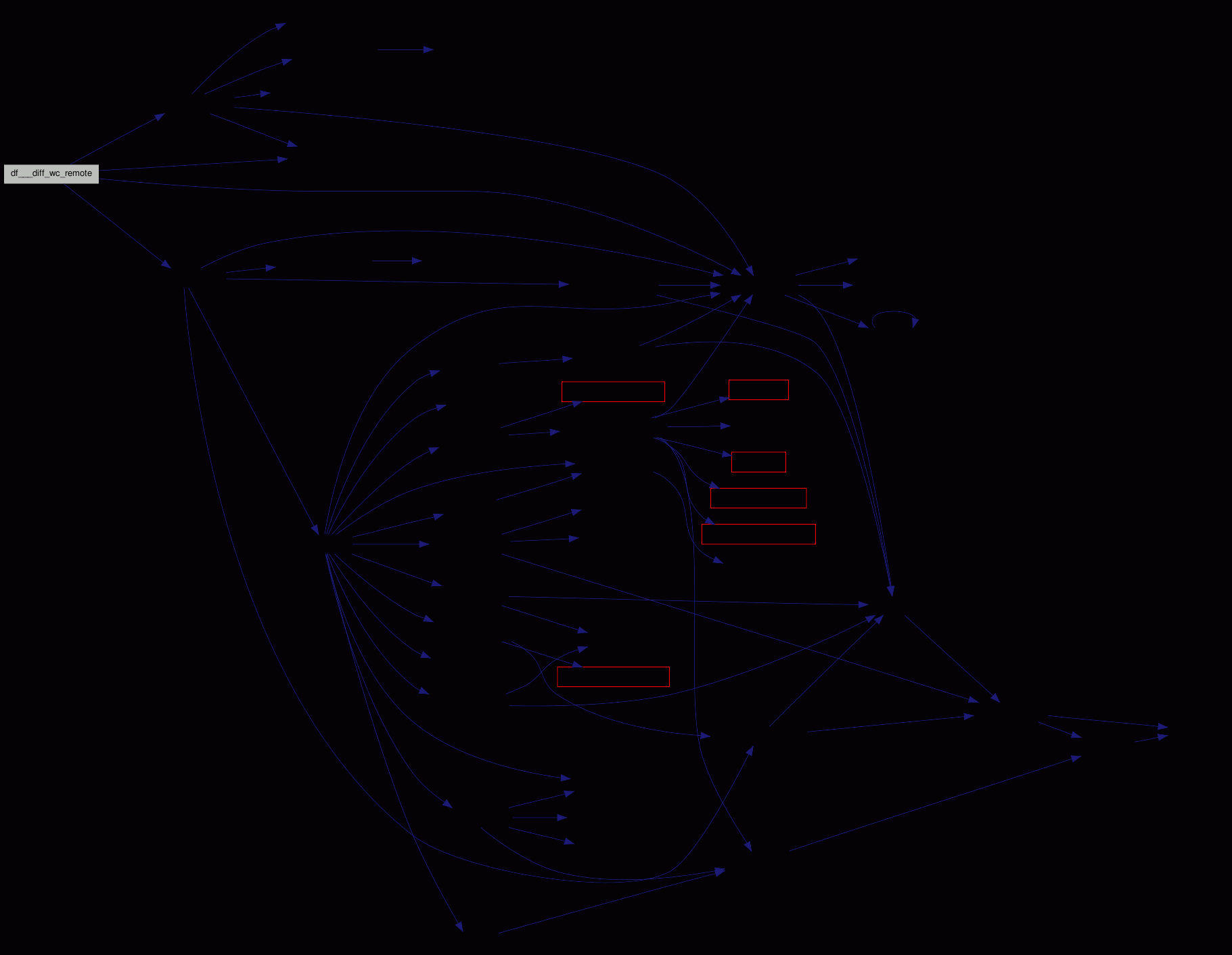Here is the caller graph for this function: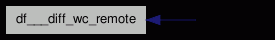int df___direct_diff ( struct estat * sts )

-.

Definition at line 515 of file diff.c.

Referenced by df__work().

Here is the call graph for this function: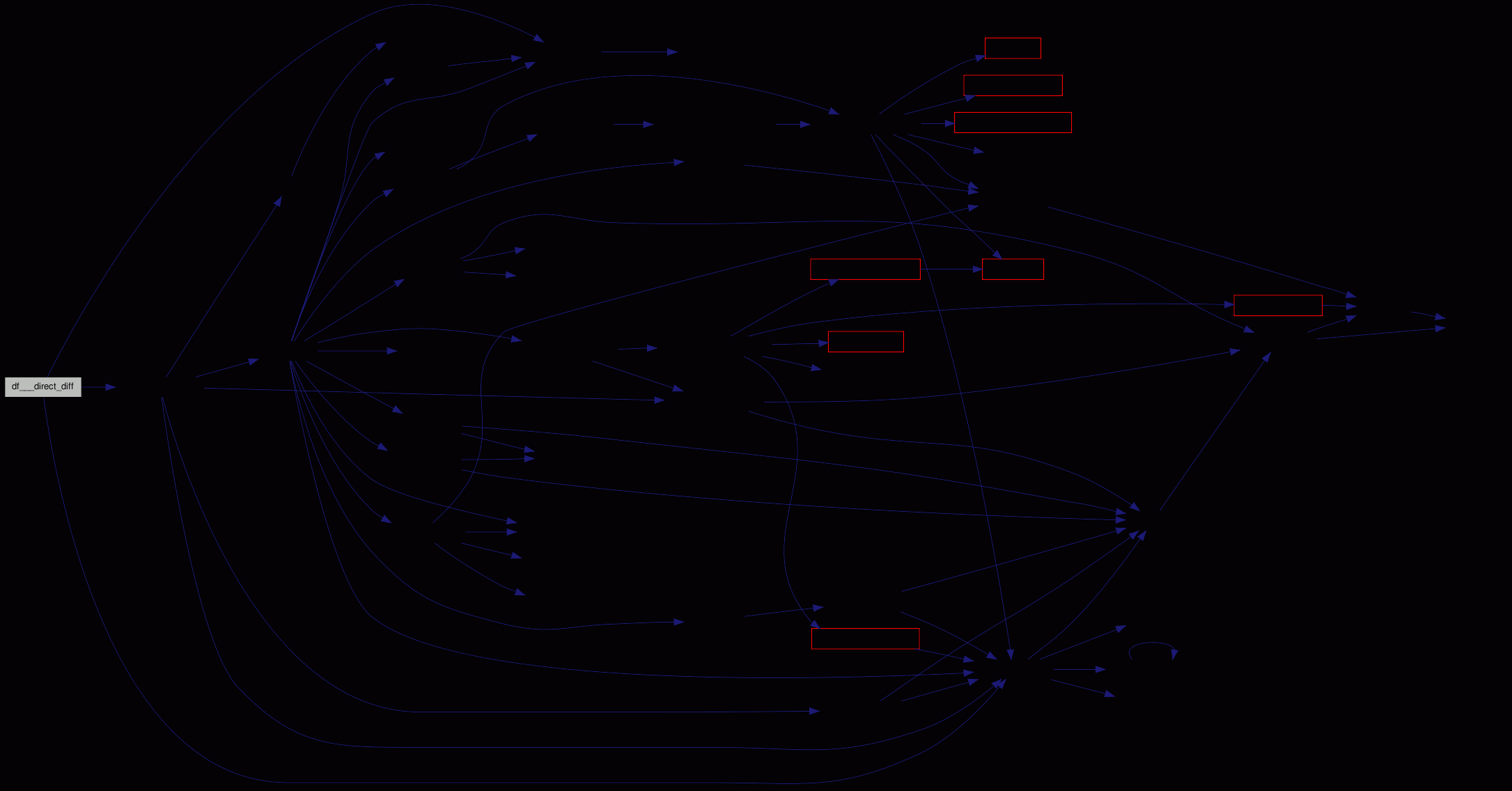Here is the caller graph for this function: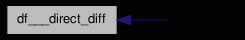int df___print_meta ( char * format, ... )

Diff the given meta-data.

The given format string is used with the va-args to generate two strings. If they are equal, one is printed (with space at front); else both are shown (with '-' and '+'). The delimiter between the two argument lists is via META_DIFF_DELIMITER. (NULL could be in the data, eg. as integer `0`.)

It would be faster to simply compare the values given to `vsnprintf()`; that could even be done here, by using two `va_list` variables and comparing. But it's not a performance problem.

Definition at line 108 of file diff.c.

References BUG_ON, DEBUGP, META_DIFF_DELIMITER, META_DIFF_MAXLEN, STOPIF_CODE_EPIPE, and STOPIF_CODE_ERR.

Referenced by df__do_diff().

Here is the caller graph for this function: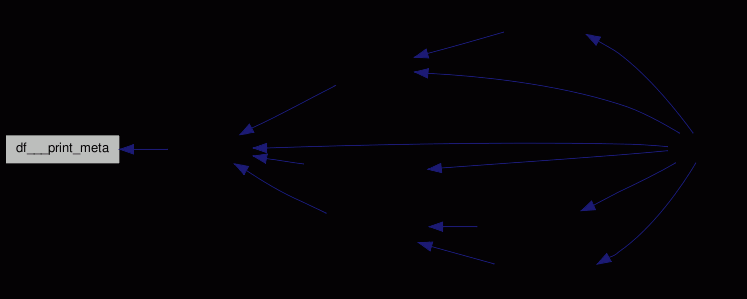int df___repos_repos ( struct estat * sts )

Does a repos/repos diff.

Currently works only for files.

Definition at line 817 of file diff.c.

Referenced by df__work().

Here is the call graph for this function: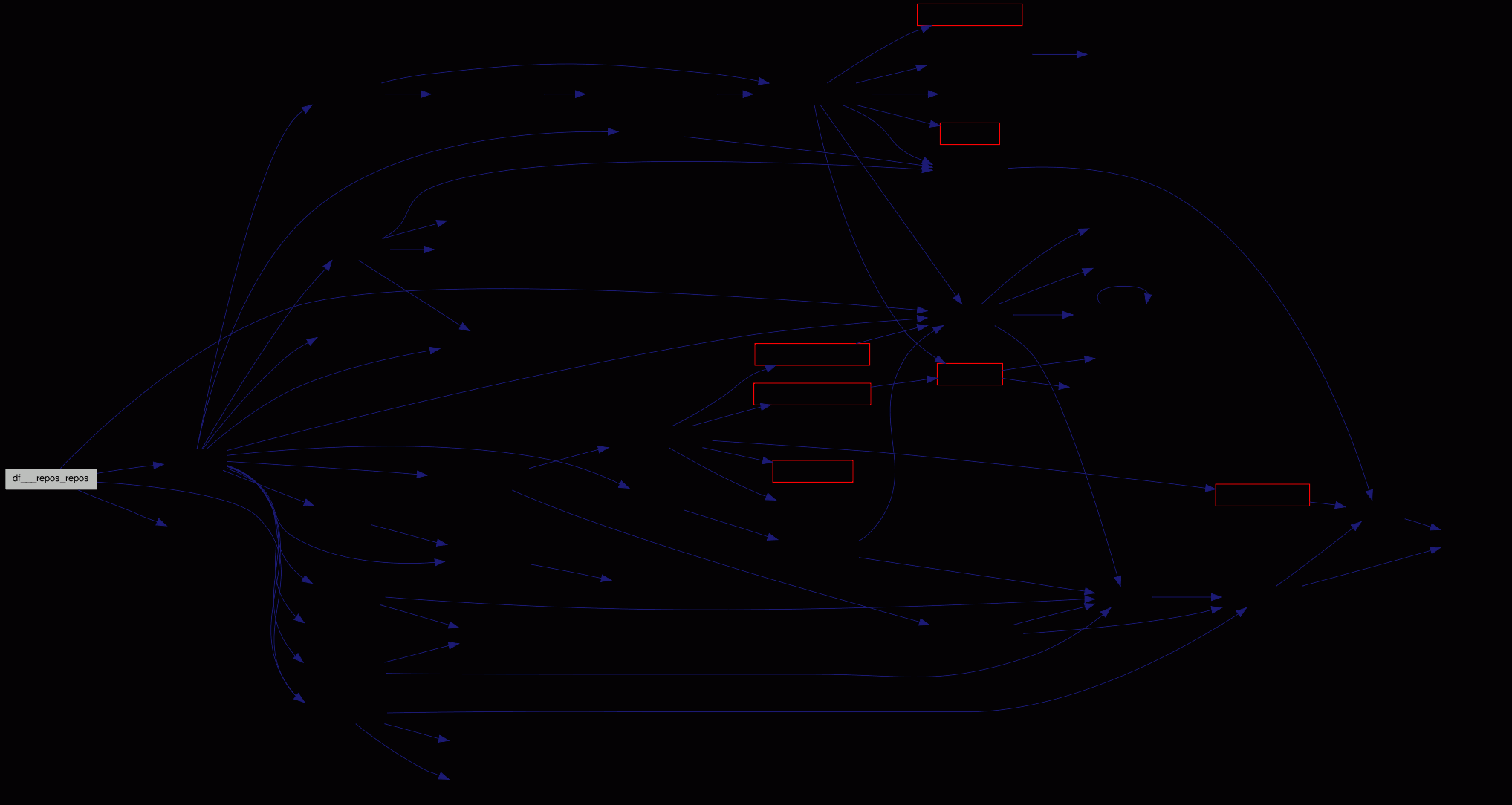Here is the caller graph for this function: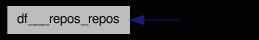int df___reset_remote_st ( struct estat * sts )

Set the entry as BASE (has no changes).

Definition at line 808 of file diff.c.

References estat::remote_status.

Referenced by df__work().

Here is the caller graph for this function: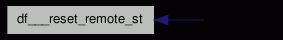static void df___signal ( int sig ) ` [static]`

FSVS GCOV MARK: df___signal should not be executed.

Signal handler function. If the user wants us to quit, we remove the temporary files, and exit.

Is there a better/cleaner way?

Definition at line 448 of file diff.c.

References DEBUGP, and df___cleanup().

Referenced by df__work().

Here is the call graph for this function: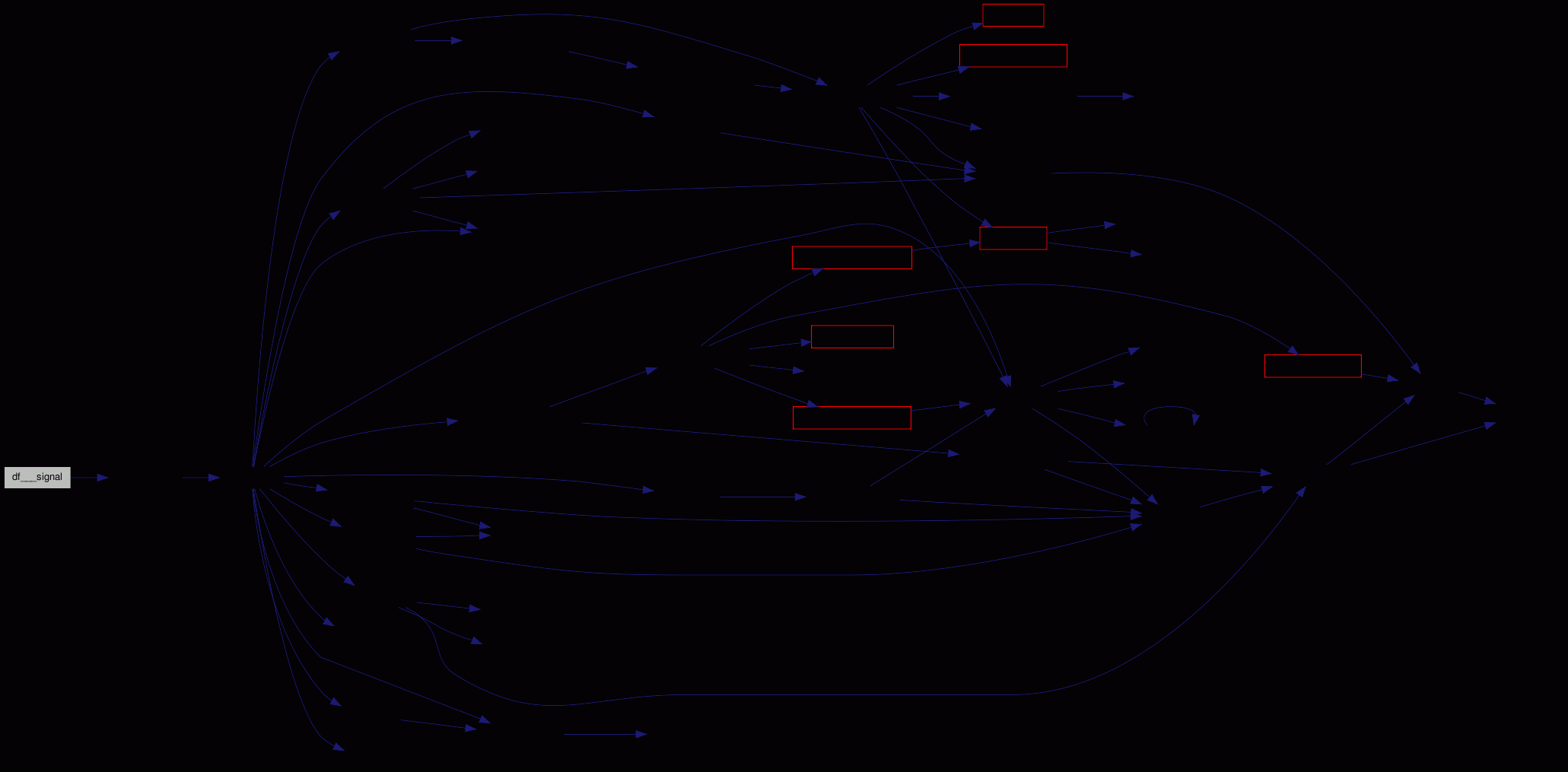Here is the caller graph for this function: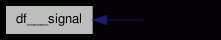int df___type_def_diff ( struct estat * sts, svn_revnum_t rev, apr_pool_t * pool )

Does a diff of the local non-directory against the given revision.

Definition at line 458 of file diff.c.

Referenced by df___diff_wc_remote(), and df___direct_diff().

Here is the call graph for this function: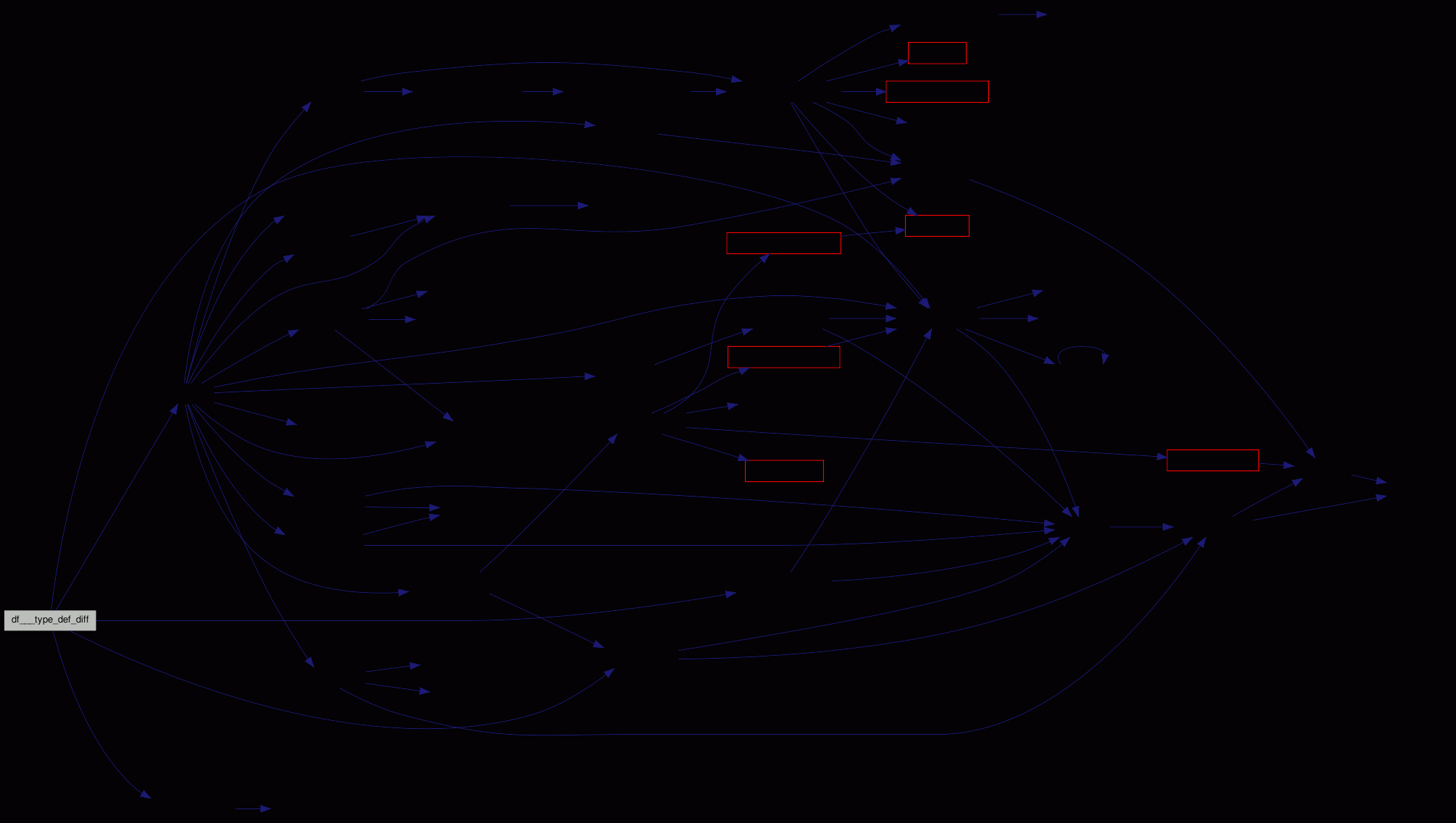Here is the caller graph for this function: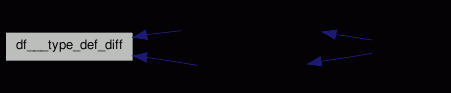int df__do_diff ( struct estat * sts, svn_revnum_t rev1, svn_revnum_t rev2, char * rev2_file )

Get a file from the repository, and initiate a diff.

Normally `rev1 == root->repos_rev`; to diff against the base revision of the file.

If the user specified only a single revision (`rev2 == 0`), the local file is diffed against this; else against the other repository version.

rev2_file is meaningful only if rev2 is 0; this file gets removed after printing the difference!

Definition at line 162 of file diff.c.

Referenced by df___cleanup(), df___repos_repos(), df___type_def_diff(), and df__work().

Here is the call graph for this function: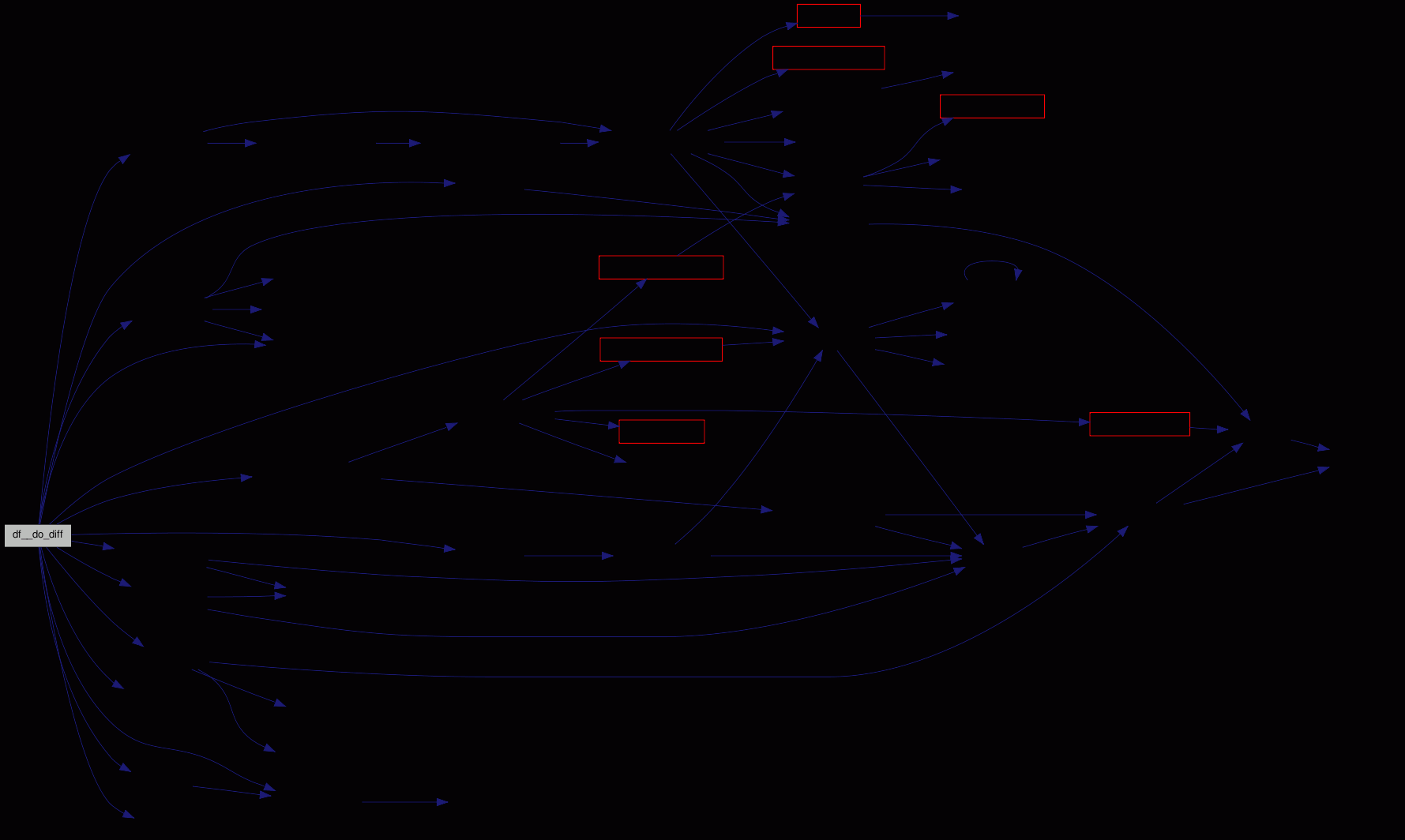Here is the caller graph for this function: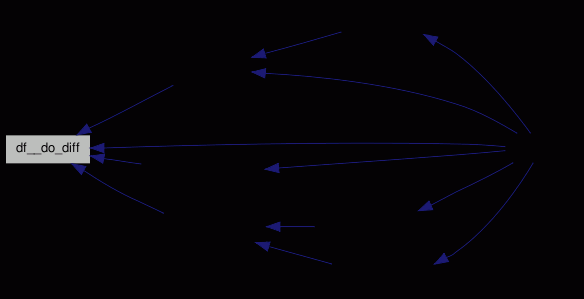int df__work ( struct estat * root, int argc, char * argv[] )

-.

We get the WC status, fetch the named changed entries, and call an external diff program for each.

As a small performance optimization we do that kind of parallel - while we're fetching a file, we run the diff.

Definition at line 887 of file diff.c.

Here is the call graph for this function: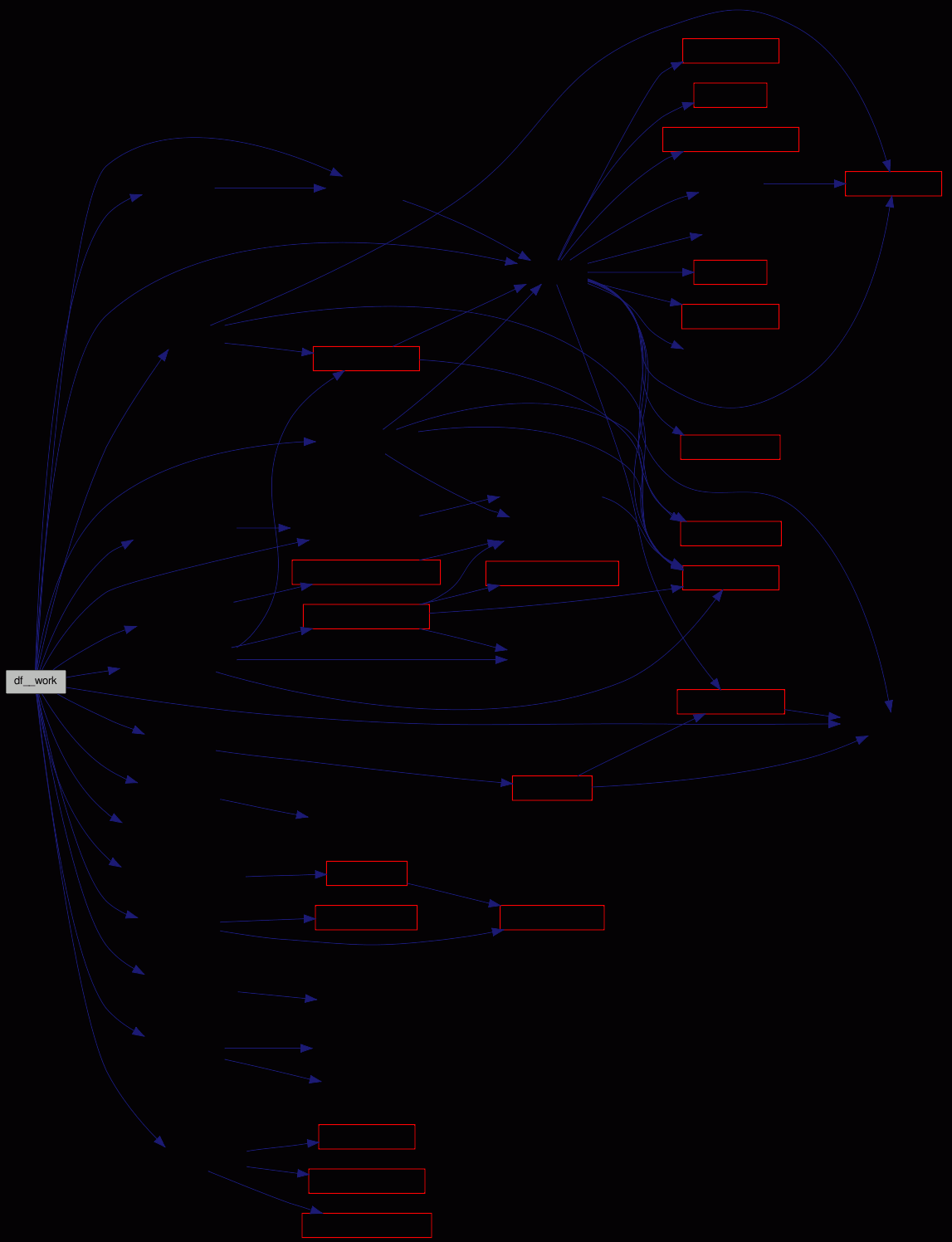## Variable Documentation

 pid_t cdiff_pid = 0

Definition at line 88 of file diff.c.

Referenced by df___cleanup(), and df__work().

 int cdiff_pipe = STDOUT_FILENO

Definition at line 87 of file diff.c.

Referenced by df___cleanup(), df__do_diff(), and df__work().

Generated for fsvs by1.6.1